Students can download Maths Chapter 5 Coordinate Geometry Ex 5.3 Questions and Answers, Notes, Samacheer Kalvi 9th Maths Guide Pdf helps you to revise the complete Tamilnadu State Board New Syllabus, helps students complete homework assignments and to score high marks in board exams.

## Tamilnadu Samacheer Kalvi 9th Maths Solutions Chapter 5 Coordinate Geometry Ex 5.3

Question 1.
Find the mid-points of the line segment joining the points.
(i) (-2, 3) and (-6, -5)
(ii) (8, -2) and (-8, 0)
(iii) (a, b) and (a + 2b, 2a – b)
(iv) ($$\frac{1}{2},\frac{-3}{7}$$) and ($$\frac{3}{2},\frac{-11}{7}$$)
Solution: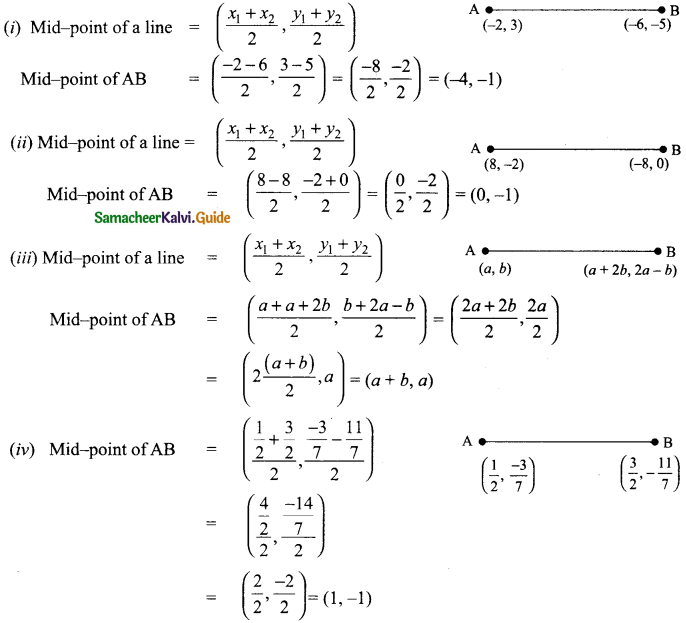Question 2.
The centre of a circle is (-4, 2). If one end of the diameter of the circle is (-3, 7) then find the other end.
Solution:
Let the other end of the diameter B be (a, b)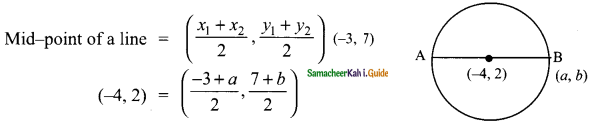∴ $$\frac{-3+a}{2}$$ = -4
-3 + a = -8
a = -8 + 3
a = -5
$$\frac{7+b}{2}$$
7 + b = 4
b = 4 – 7 ⇒ b = -3
The other end of the diameter is (-5, -3).Question 3.
If the mid-point (x, y) of the line joining (3, 4) and (p, 7) lies on 2x + 2y + 1 = 0, then what will be the value of p?
Solution: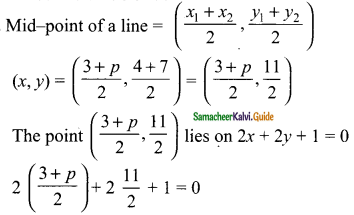3 + p + 11 + 1 = 0 ⇒ p + 15 = 0 ⇒ p = -15
The value of p is -15.Question 4.
The mid-point of the sides of a triangle are (2, 4), (-2, 3) and (5, 2). Find the coordinates of the vertices of the triangle.
Solution:
Let the vertices of the ΔABC be A (x1 y1), B (x2, y2) and C (x3, y3)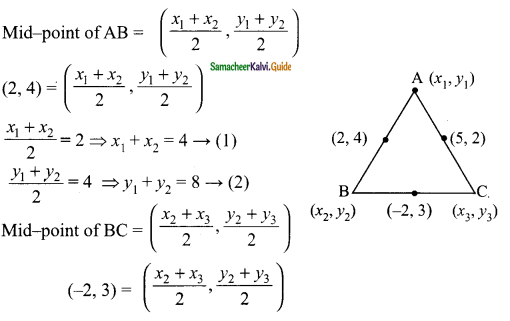$$\frac{x_{2}+x_{3}}{2}$$ = -2 ⇒ x2 + x3 = -4 → (3)
$$\frac{y_{2}+y_{3}}{2}$$ = 3 ⇒ y2 + y3 = 6 → (4)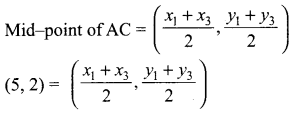$$\frac{x_{1}+x_{3}}{2}$$ = 5 ⇒ x1 + x3 = 10 → (5)
$$\frac{y_{1}+y_{3}}{2}$$ = 2 ⇒ y1 + y3 = 4 → (6)
By adding (1) + (3) + (5) we get
2x1 + 2x2 + 2x3 = 4 – 4 + 10
2(x1 + x2 + x3) = 10 ⇒ x1 + x2 + x3 = 5
From (1) x1 + x2 = 4 ⇒ 4 + x3 = 5
x3 = 5 – 4 = 1
∴ The vertices of the ΔABC are
A (9, 3)
B (-5, 5), C (1, 1)
From (3) x2 + x3 = -4 ⇒ x1 + (-4) = 5
x1 = 5 + 4 = 9
From (5) ⇒ x1 + x3 = 8
x2 + 10 = 5
x2 = 5 – 10 = -5
∴ x1 = 9, x2 = -5, x3 = 1
By adding (2) + (4) + (6) we get
2y3 + 2y2 + 2y3 = 8 + 6 + 4
2(y1 +y2 + y3) = 18 ⇒ y1 + y2 + y3 = 9
From (2) ⇒ y1 + y2 = 8
8 + y3 = 9 ⇒ y3 = 9 – 8 = 1
From (4)
y2 + y3 = 6 ⇒ y1 + 6 = 9
y1 = 9 – 6 = 3
From (6)
y1 + y3 = 4 ⇒ y2 + 4 = 9
y2 = 9 – 4 = 5
∴ y1 = 3, y2 = 5, y3 = 1Question 5.
O (0, 0) is the centre of a circle whose one chord is AB, where the points A and B (8, 6) and (10, 0) respectively. OD is the perpendicular from the centre to the chord AB. Find the coordinates of the mid-point of OD.
Solution:
Note: Since OD is perpendicular to AB, OD bisect the chord
D is the mid-point of AB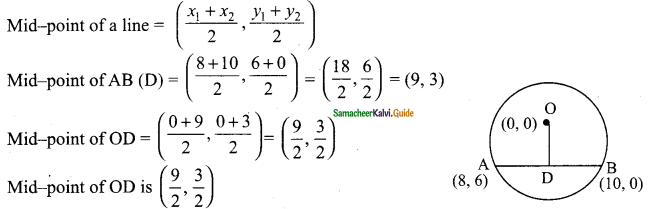Question 6.
The points A(-5, 4) , B(-1, -2) and C(5, 2) are the vertices of an isosceles right-angled triangle where the right angle is at B. Find the coordinates of D so that ABCD is a square.
Solution:
Since ABCD is a square
Mid-point of AC = mid-point of BD
Let the point D be (a, b)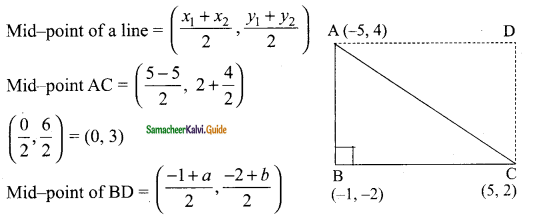But mid-point of BD = Mid-point of AC
($$\frac{-1+a}{2}, \frac{-2+b}{2}$$) = (0, 3)
$$\frac{-1+a}{2}$$ = 0
-1 + a = 0
a = 1
($$\frac{-2+b}{2}$$)
-2 + b = 6
b = 6 + 2 = 8
∴ The vertices D is (1, 8).Question 7.
The points A (-3, 6), B (0, 7) and C (1, 9) are the mid points of the sides DE, EF and FD of a triangle DEF. Show that the quadrilateral ABCD is a parallellogram.
Solution:
Let D be (x1 y1), E (x2, y2) and F (x3, y3)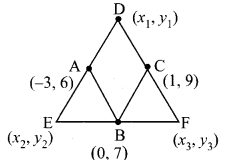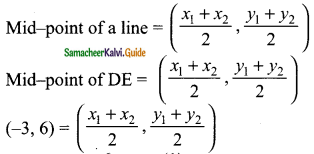x1 + x2 = -6 → (1)
y1 + y2 = 12 → (2)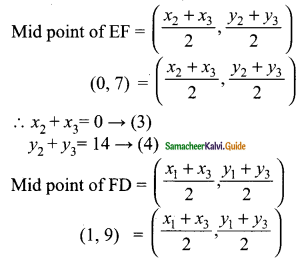x1 + x3 = 2 → (5)
y1 + y3 = 18 → (6)
Add (1) + (3) + (5)
2x1 + 2x2 + 2x3 = -6 + 0 + 2
2 (x1 + x2 + x3) = -4
x1 + x2 + x3 = -2
From (1) x1 + x2 = -6
x3 = -2 + 6 = 4
From (3) x2 + x3 = 0
x1 = -2 + 0
x1 = -2
From (5) x1 + x3 = 2
x2 + 2 = -2
x2 = -2 -2 = -4
∴ x1 = -2, x2 = -4, x3= 4
Add (2) + (4) + 6
2y1 + 2y2 + 2y3 = 12 + 14 + 18
2(y1 + y2 + y3) = 44
y1 + y2+ y3 = 44/2 = 22
From (2) y1 + y2 = 12
12 + y3 = 22 ⇒ y3 = 22 – 12 = 11
From (4) y2 + y3 = 14
y1 + 14 = 22 ⇒ y1 = 22 – 14
y1 = 8
From (6)
y1 +y3 = 18
y2 + 18 = 22 ⇒ = 22 – 18
= 4
∴ y1 = 8, y2 = 4, y3 = 11
The vertices D is (-2, 8)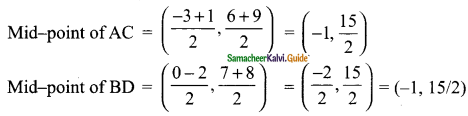Mid-point of diagonal AC = Mid-point of diagonal BD
The quadrilateral ABCD is a parallelogramQuestion 8.
A(-3, 2) , B(3, 2) and C(-3, -2) are the vertices of the right triangle, right angled at A. Show that the mid point of the hypotenuse is equidistant from the vertices.
Solution: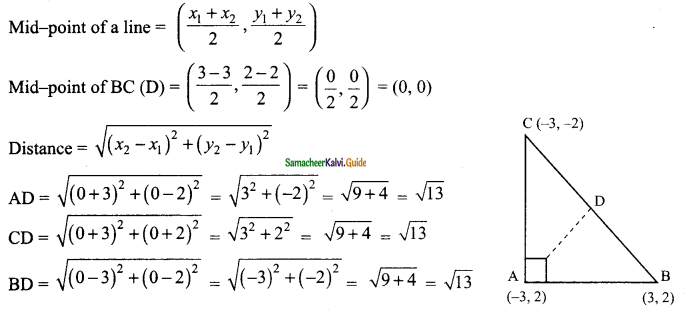AD = CD = BD = $$\sqrt{13}$$
Mid-point of BC is equidistance from the centre.